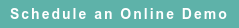# RoyaltyStat Blog

### Posts by Topic

We discuss three contending transfer pricing models. Let P = “Net” profits, S = Sales, and A = Assets: For simplicity, we don’t consider profit markup on total costs, and exclude random errors:

(1)  P(i) = α0 + α1 S(i), which is a model of profit margin.

(2)  P(i) = β0 + β1 A(i), which is a model of profit rate (in OECD lingo: “return on assets”).

(3)  P(i) = γ0 + γ1 S(i) + γ2 A(i), which is a model of adjusted profit margin (profit margin adjusted for asset turnover or profit margin adjusted for asset instensity).

These rival profit models can be run using OLS (ordinary least squares) and data from i = 1, 2, …, N comparable uncontrolled enterprises.

One way to select the best NPI is to run three regression models and select the model with the smallest standard error. However, OLS is not abiding and if Revenue and Assets are correlated, we must use model (3) because otherwise α1 or β1 is biased; and biased coefficients are impermissible in science.

To measure this bias, we test the correlation between Revenue and Assets:

(4)  A(i) = δ0 + δ1 S(i), which is asset turnover (“asset intensity”).

Empirical tests using Capital IQ Compustat enterprise-level data in RoyaltyStat shows that estimates of δ1 ≈ 0.8, which is high positive correlation between revenue and assets.

We substitute (4) into (3) and obtain:

(5)  P(i) = γ0 + γ1 S(i) + γ0 + δ1 S(i)}

= γ0 + γ2 δ0 + γ1 S(i) + γ2 δ1 S(i)

= λ0 + λ1 S(i)

where λ0 = γ0 + γ2 δ0 and λ1 = γ1 + γ2 δ1.

Above, the factor γ2 δ1 is a measure of bias if we use profit model (1) and δ1 is different from zero. We can find the bias using model (2) in similar fashion.

Therefore, we must use model (3) when δ1 is significant, not zero. Else, we can use (1) or (2) when δ1 = 0, or when δ1 is insignificant or negligible (immaterial).

Selecting the most appropriate NPI is not simple like the role of Paladin in Charlemagne’s court. To be defensible against audit scrutiny, we must use statistical analysis to select an unbiased NPI that has the smallest standard error among competing unbiased profit indicators.

Published on Oct 25, 2018 9:39:47 PM

Ednaldo Silva (Ph.D.) is founder and managing director of RoyaltyStat. He helped draft the US transfer pricing regulations and developed the comparable profits method called TNNM by the OECD. He can be contacted at: esilva@royaltystat.com

RoyaltyStat provides premier online databases of royalty rates extracted from unredacted license agreements
and normalized company financials (income statement, balance sheet, cash flow). We provide high-quality data, built-in analytical tools, customer training and attentive technical support.Topics: Net Profit Indicator